## Example Questions

2 Next →

### Example Question #21 : Prisms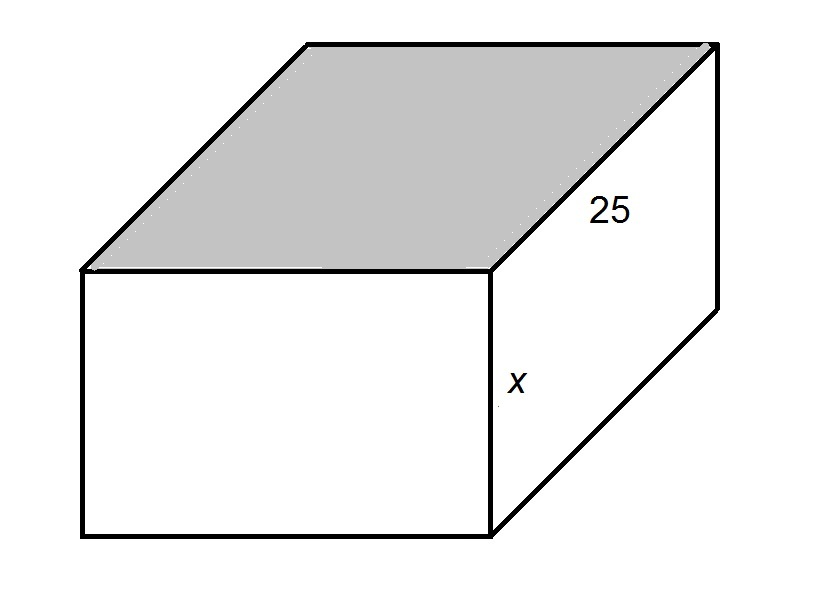The shaded face of the above rectangular prism is a square. In terms of, give the surface area of the prism.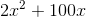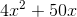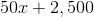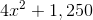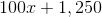Explanation:

Since the top face of the prism is a square, the common sidelength - and the missing dimension - is 25.

The surface areaof a rectangular prism with length, width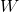, and height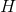can be found using the formula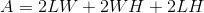.

Setting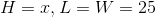, and solving for: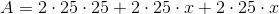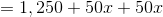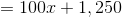2 Next →